# NCERT Solution Exercise – 6.2 Class 10

Q.1 In fig.6.17,(i)and(ii),DE || BC. find EC in(i)and AD in (ii)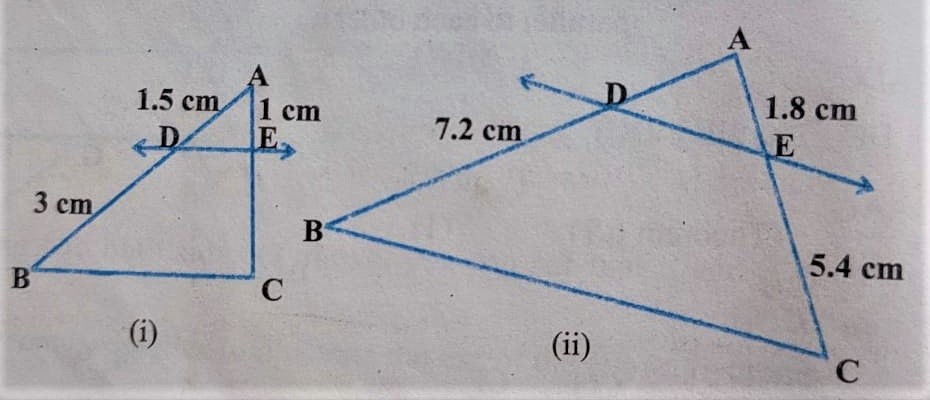(i)

Solution:

According to figure (i) in ΔABC

DE || BC { given }

By B.P.T. [ In a triangle if a line is parallel to one side divides remaining to sides in equal ratio ]

AD / BD = AE / EC

1.5/3 = 1/EC

⇒1.5EC = 3

⇒ EC = 3/1.5

⇒ EC = 30/15

⇒ EC = 2cm

(ii)Solution:

According to Figure (ii)

in ΔABC

DE || BC  { given }

by B.P.T. [ In a triangle if a line is parallel to one side, divide the remaining two sides in an equal ratio ]

Q.2 E and F are points on the sides of PQ and PR respectively of a Δ PQR. For each of the following cases , state whether

EF || QR.

(i) PE = 3.9cm, EQ = 3cm, PE = 3.6cm and FR = 2.4cm

Solution:

PE/QE = PE/FR

⇒ 3.9/3×10 = 3.6×10/2.4×10

⇒ 13/10 ≠ 3/2 [ Sides of ration are not equal ]

Hence EF is not || to QR.

(ii) PE=4cm, QR=4.5cm, PF=8cm and RF=9cm

Solution:

PE/QE = PF/RF

⇒ 4/4.5 ≠ 8/9 [ Sides of ration are not equal ]

⇒ Hence EF is not parallel to QR

(iii) PQ= 1.28cm, PR=2.56cm, PE=0.18cm and  PF=0.36cm.

Solution:

IF PQ/PE = PR/PF

⇒ 1.28/0.18 = 2.56/0.36

⇒ 128×100/18×100 = 256×100/36×100

⇒ 128/18 = 256/36

⇒ 64/9 = 64/9 [ Sides of ratio are not equal ]

by  Converse of BPT

EF is Parallel to QR

Q.3 In fig. 6.18 if LM || CB and LN || CD, prove that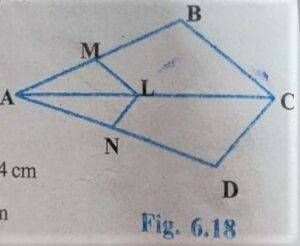Solution:

According to Figure:-

In Δ ABC

LM || CB { given }

BY B.P.T. [ In a triangle if a line is parallel to one side divide the remaining two sides in an equal ratio ]

AM/AB = AL/AC ———(i)

Similarly

LN || CD

By B.P.T.

Hence proved

Q.4 In fig. 6.19, DE || AC and DF || AE Prove that BF/FE = BE/EC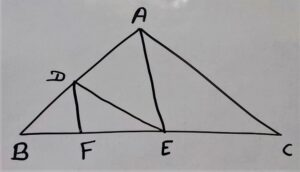Solution:

In Δ ABC

DE || AC

BD/AD = BE/CE [∴by B.P.T.] ——–(i)

Similarly

In Δ ABE

DF || AE

BD/AD = BF/EF ———(ii) [ by B.P.T ]

by comparing equation (i) and equation(ii)

We get

BE/CE = BF/EF

⇒ BE/EC = BF/FE

Hence Proved

Q.5 In fig. 6.20, DE || OQ and DE || OR. show that EF || QR.

Given:DE || OQ

DE || OR

To Show:

EF II QR

Solution:

In ΔPOQ

DE || OQ

PE/EQ = PD/DO ———(i) [ ∴ by B.P.T. ]

In ΔPOR

DF || OR

PE/FR = PD/DO——–(ii) [∴ by BPT ]

by equation (i) and  equation (ii)

PE/EQ = PF/FR

By converse of BPT

EF || OR

equation (i) and equation (ii)

Q.6 In Fig. 6.21 A, B, and C  are points on OP, OQ, and OR respectively such that AB || PQ and AC || PR Show that BC || QR

Given: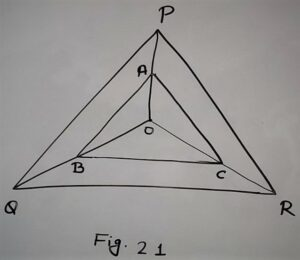A, B, and C are lies on sides OP, OQ, and OR respectively

AB || PQ,

AC || PR

Solution:

In Δ PQR

AB || PQ [∴given]

by B.P.T.

PA/AO = QB/BO ———-(i)

Similarly

In Δ POR

AC || PR [∴ given ]

by B.P.T.

PA/AO = RC/CO ———-(ii)

Q.7 Using Theorem 6.1, prove that a line drawn through the mid-point of one side of a triangle parallel to another side bisects the third side.

Given: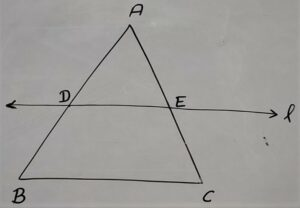In ΔABC

D is the midpoint of side AB

BC II Line l [BC parallel to line l]

Line l intersects AC at E.

To Prove:

E, Mid-point of AC

Proof:

Here According to the figure

In ΔABC

D is the mid-point of AB

DE II BC [Given]

∴ AD/DB = AE/EC  [By BPT]

⇒ 1 = AE/EC [From equation (i)]

∴ AE = EC

∴ E is the mid-point of AC.

Q.8 Using Theorem 6.2, prove that the line joining the mid-points of any two sides of a triangle is parallel to the third side.

Given:

In ΔABC

D = Mid-point of AB

E = Mid-point of AC

Join D to E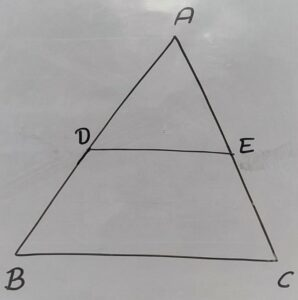To Prove:

DE II BC

Proof:

According to Figure

In ΔABC

D is the mid-point of AB  [given]

E is the Mid-point of AC [Given]

∴ AE=EC

⇒ AE/EC=1 ……………………….(ii)

From Equation(i) and Equation(ii)

We get

By Converse of BPT

DE II BC

Hence Proved.

Q.9 ABCD is a trapezium in which AB || DC and its diagonals intersect each other at point O. Show that

AO/BO = CO/DO

Given:

In trapezium ABCD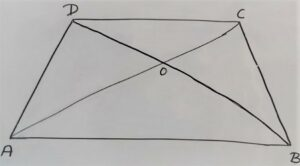AB || DC

To Show:

AO/BO = CO/DO

Construction:

EO || AB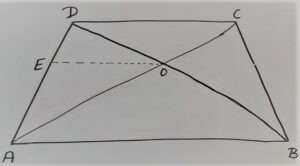Proof:

In Δ ABD

EO || AB [∴by const.]

By BPT

AE/ED = BO/OD ———(i)

In Δ ACD

AB || DC [∴ given ]

EO || AB [∴ By construction ]

∴ DC || EO

AE/ED = AO/CO ——-(ii)

by comparing equation(i) and equation(ii)

BO/OD = AO/CO

⇒ CO/DO = AO/BO

⇒ AO/BO = CO/DO

Hence Proved

Q.10 The diagonals of a quadrilateral ABCD intersect each other at point  O such that AO/BO = CO/DO. Show that  ABCD is a trapezium.

Given:AO/BO = CO/DO

⇒ AO/CO = BO/DO ——–(i)

To Show:

ABCD is a trapezium

Construction:

EO || ABProof:

In Δ ABD

EO || AB [∴by construction]

by B.P.T.

AE/DE = BO/DO ———(ii)

by comparing equation(i) and equation(ii)

We get

AO/CO = AE/DE ——-(iii)

In Δ ACD

AO/CO = AE/DE [∴From Equation (iii) ]

By the converse of BPT

EO || DC

∴ EO || AB [∴ by construction]

∴ AB || DC

Hence pair of one side are parallel of Quadrilateral ABCD .

∴ Quadrilateral ABCD is a trapezium .

Hence proved.